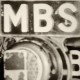# Week 2- Off To A Great Start

Another week done another batch of work done here’s everything I have for this week

Tutorials

Problem set

Blog questions

How can you use “falsey-ness” to determine if a string value can be coerced to a number? Why does this work? What is “type coercion” anyway?

falsey-ness is used to test if a value is true or not in the console. the type coercion is to coerce a number to a string and a string to a number.

``````!Number(str) //to test if that's a number
Falsy Values
false
""
null
undefied
NaN
0``````

What do you need to do to convert a for loop to a while loop?

A for loop has an initializer, condition, and increment-er. A while loop only has a condition.

``````i = 1 ;
while i <=3
j = 2 ;
while j <=4
A(i,j) = B(i+2,j+1) ;
j = j+1 ;
end
i = i+1 ;
end``````

## Join the Conversation

1.1 Comment

1.admin says:

Hey let’s get those lovely blogging questions answered!

And generally good work with the problem set. I did note you have an infinite loop in the ‘fizz buzz’ exercise. Eek! It’s due to a typo in your `for` loop conditions. There is a ‘1’ instead of an ‘i’ in the while condition.

`for(i = 1; 1 < 101; i++)`
```for(i = 1; i < 101; i++)
```